# 第10周

## 十七、大规模机器学习(Large Scale Machine Learning)

### 17.1 大型数据集的学习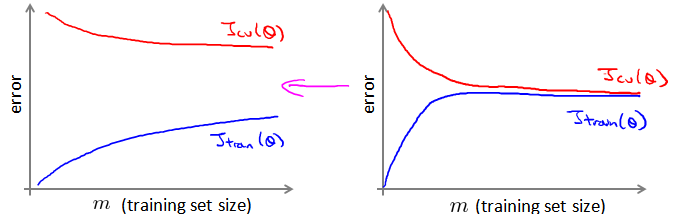### 17.2 随机梯度下降法

$cost\left( \theta, \left( {x}^{(i)} , {y}^{(i)} \right) \right) = \frac{1}{2}\left( {h}_{\theta}\left({x}^{(i)}\right)-{y}^{{(i)}} \right)^{2}$

for $i = 1:m${

$\theta:={\theta}_{j}-\alpha\left( {h}_{\theta}\left({x}^{(i)}\right)-{y}^{(i)} \right){{x}_{j}}^{(i)}$

​ (for $j=0:n$)

​ } }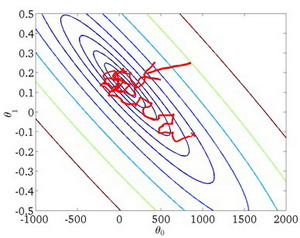### 17.3 小批量梯度下降

for $i = 1:m${

$\theta:={\theta}_{j}-\alpha\frac{1}{b}\sum_\limits{k=i}^{i+b-1}\left( {h}_{\theta}\left({x}^{(k)}\right)-{y}^{(k)} \right){{x}_{j}}^{(k)}$

​ (for $j=0:n$)

$i +=10$

​ } }

### 17.4 随机梯度下降收敛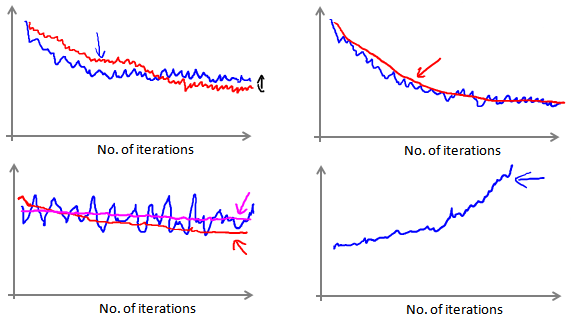$\alpha = \frac{const1}{iterationNumber + const2}$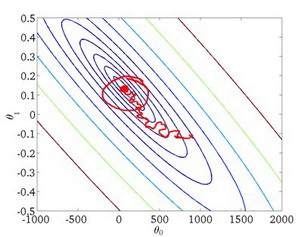### 17.6 映射化简和数据并行## 十八、应用实例：图片文字识别(Application Example: Photo OCR)

### 18.1 问题描述和流程图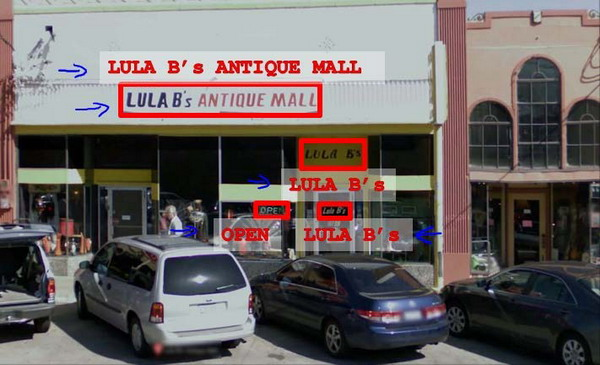1. 文字侦测（Text detection）——将图片上的文字与其他环境对象分离开来
2. 字符切分（Character segmentation）——将文字分割成一个个单一的字符
3. 字符分类（Character classification）——确定每一个字符是什么 可以用任务流程图来表达这个问题，每一项任务可以由一个单独的小队来负责解决：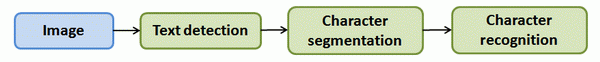### 18.2 滑动窗口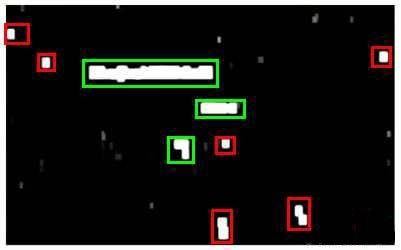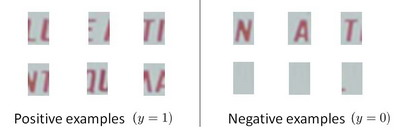1. 人工数据合成
2. 手动收集、标记数据
3. 众包

### 18.4 上限分析：哪部分管道的接下去做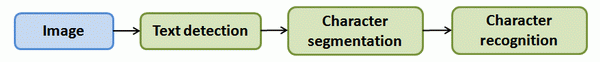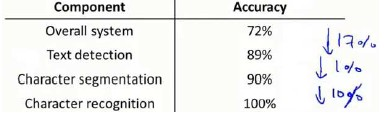Andew Ng